# 「語学」は、耳から!!

mail: info@gaigo.co.jp　 tel: 03-5646-5324

41 数学 / 42 物理学 / 50 技術・工学

# 物理の数学 びっくり箱 全15巻

## Math in a Box 15-part series

• 規　　格: DVD(英語音声/英語字幕表示機能付き) 全15巻(各巻22分～59分)
英文チャプタガイド付き
• 制　　作: 米国FFH 1999年
• 本体価格: 15巻セット 240,000円　分売各巻 18,000円
• 注文番号: GLS-029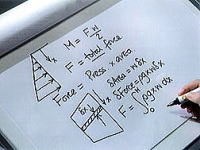Too many students view mathematics as merely a hurdle to be jumped in their race for an education rather than as a fundamental tool for understanding and solving scientific and mechanical problems. Using real-world examples—plus plenty of animation and graphics—this comprehensive 15-part series helps students to visualize the relationship between forces and motion through phenomena that characterize them. Each program is presented by Dr. Timothy David, a mathematician at the University of Leeds’ School of Engineering, and consists of two sections: a case study, with a series of questions and a hint as to how to construct the mathematical model, and a detailed explanation of the principles behind the case study, along with the solutions to the equations. 15-part series, 21-58 minutes each.

###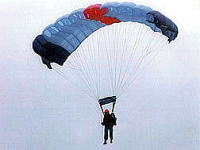01 落下の数学: 重力・重量・質量・浮力・加速度

###### Parachuting: Moving Bodies with Constant Mass
This program uses a parachutist to demonstrate the effects of drag on the force of gravity, showing how to make mathematical approximations and how the resultant forces can be equated to the product of mass and acceleration. A first-order differential equation is then used to find the minimum height from which a parachutist can jump without injury. (21 minutes)

###02 空へ、宇宙へ: 推進力の数学

###### Take-off: Moving Bodies with Constant Mass
This program shows that a single mathematical model can describe the take-off of a wide variety of aircraft, running the gamut from a single-engine trainer to the Concorde. Because the search for approximations is somewhat complex, graphical notation is employed to reveal the interaction and variation of the forces involved. (26 minutes)

###03 飛行の数学: 弓と矢・エアクラフト

###### Bows, Arrows, and Aircraft Carriers: Moving Bodies with Constant Mass
In this program, geometry is combined with approximation to solve relatively complex problems involving shooting an arrow and landing an airplane on the deck of an aircraft carrier. Emphasizing the value of sketching as a visualization tool, the program also explains how the solution of the archery problem, through geometric inversion, can help solve the problem of a plane landing. (59 minutes)

###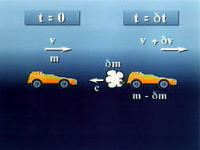04 推進力の数学: 変動質量の場合

###### Impulse: Moving Bodies with Variable Mass
Using Newton’s second law and a balloon-powered toy car, this program examines how impulse relates to the change in momentum and how the rate of that change equates to the resultant force. In addition, the exhaust velocity of the jet-propelled car is estimated. (39 minutes)

###05 ロケットや雪崩で学ぶ変動質量の数学

###### Rockets and Avalanches: Moving Bodies with Variable Mass
In this program, footage of rockets blasting off and avalanches roaring down mountainsides provides two perspectives of the same principle: the rate of change of momentum. Practical calculations of the time it takes for a rocket to lift off and the time a skier has to escape a landslide, plus an experiment with eggs and flour, reinforce the lesson. (42 minutes)

###06 ベクトルの数学: 基本

###### Modeling Vectors
Employing diverse examples such as trains and water slides, this program illustrates the use of vectors to represent forces operating in both two and three dimensions. The algebraic manipulation of vectors in modeling problems is featured. (24 minutes)

### 07 ベクトルの数学: 凧の原理

######Kies: Modeling with Vectors 28
After defining the basic concepts of vectors, this program uses algebra to determine how the resultant of numerous forces acting on a body can be obtained and then equated to the product of mass and acceleration. Kites are employed to exemplify both equilibrium and non-equilibrium conditions. (28 minutes)

###08 ベクトルの数学: 回転

###### Vectors and Moments
Moving beyond the concept of simple force, this program expands the application of vectors to include velocity, acceleration, and rotational motion. In addition, moments of greater complexity are investigated through vector geometry. Real-world examples are included. (56 minutes)

###09 転のちからと数学

###### Doors, Heart Valves, and Flic-Flacs: Moments
After explaining the principle of moments, this program shows how apparently dissimilar physical phenomena are actually mathematically similar through the examples of a synthetic heart valve, a lock gate, and the gymnastic maneuver known as a flic-flac, or back handspring. The need to make careful approximations during the modeling process is stressed. (51 minutes)

###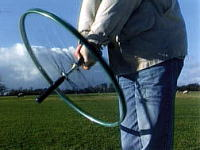10 独楽の不思議を科学する

###### Spinning Tops and Ailerons: Moments & Angular Momentum
This program discusses how geometry, gravity, and the coefficient of friction determine whether an object slides or topples. The mathematical models behind these phenomena are presented through examples such as ice hockey and skiing. The relationship between sliding and a body’s velocity as an exercise in energy conservation is explored as well. (33 minutes)

###11 滑りと摩擦の数学

###### Sliding and Toppling: Modeling Forces
This program discusses how geometry, gravity, and the coefficient of friction determine whether an object slides or topples. The mathematical models behind these phenomena are presented through examples such as ice hockey and skiing. The relationship between sliding and a body’s velocity as an exercise in energy conservation is explored as well. (33 minutes)

###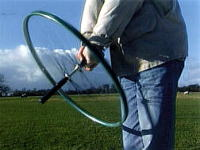12 遠心力と求心力の数学

###### Bikes and Cars: Centripetal Acceleration
This program considers the idea that circular motion must imply a force or component of a force toward the center of a circle, as in the Newtonian theory of how the Moon orbits the Earth. The reasons why bicyclists lean during turns, why roads are banked, and why car tires react as they do during a turn are investigated. (36 minutes)

###13 周期性の数学: 振り子の原理

###### Pendulum: Simple Harmonic Motion
This program introduces the concept of simple harmonic motion through the operation of the pendulum. The findings of Galileo and his contemporaries on the mechanics of the pendulum are presented, along with examples of pendular motion drawn from the modern world. (54 minutes)

###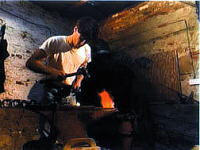14 周期性の数学: バネの原理

###### Damping: Simple Harmonic Motion
This program investigates how the mathematical model of simple harmonic motion becomes more complex through the introduction of damping. The application of simple modeling techniques to create homogeneous linear second-order differential equations is illustrated. (44 minutes)

###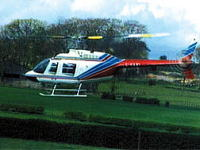15 周期性の数学: 共振・共鳴の原理

###### Resonance: Simple Harmonic Motion
In this program, resonance is examined. The value of mathematical models is demonstrated through the physics of applying a time-varying force to a body that fundamentally exhibits simple harmonic motion. Solution techniques for general linear second-order differential equations are featured. (50 minutes)

ご注文について

このため、ご利用者(ご購入者)、ならびにご利用場所について、ご注文時に確認させて頂きます。
ご注文は、お客様(ご選定の先生または事務ご担当者様)から、直接、弊社にメール・電話・FAXでご連絡をお願いたします。